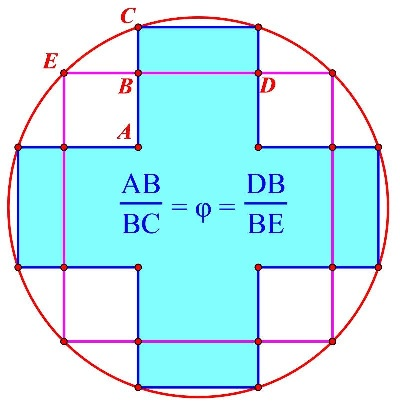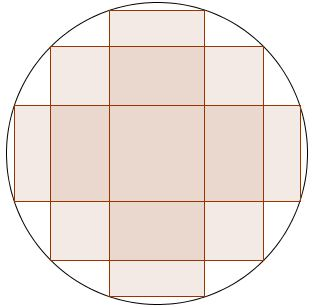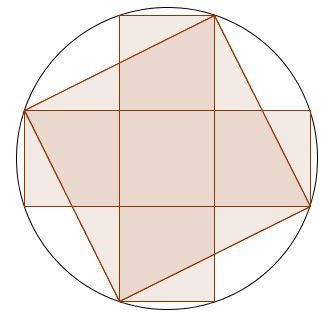# Between a Cross and a Square

The following is a new invention of Bui Quang Tuan.In the diagram the cross consists of five equal squares. Here's a more explicit depiction:Let $s$ be the side of the inscribed square, $c$ the side of any of the five squares that compose the cross. Then $s^{2}=5c^{2}$ as it follows from the diagram below (and actually has been established on another occasion):Given that $\displaystyle\frac{s}{c}=\sqrt{5}$ this is the configuration we have to investigate:So this is what we have:

\begin{align}\displaystyle \frac{DB}{BE}&=\frac{2c}{s-c}\\ &=\frac{2}{\sqrt{5}-1}\\ &=\frac{\sqrt{5}+1}{2}\\ &=\phi. \end{align}

The fraction $\displaystyle\frac{AB}{BC}$ reduces to $\displaystyle\frac{s-c}{3c-s}$ which is also shown to equal $\phi.$

The configuration at hand relates to that by Tran Quang Hung but highlights the relation between different pairs of segments.

Alexandre Borovik has observed that the rectangle with vertices $A,$ $B,$ $D$ is golden. "Between a cross and a square lies a goldfen rectangle" might be a better caption for this page.### Golden Ratio